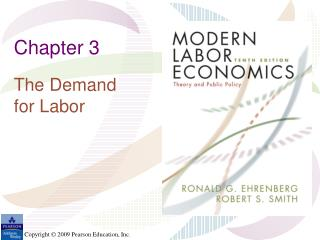DownloadDownload PresentationChapter 3

# Chapter 3

Download Presentation## Chapter 3

- - - - - - - - - - - - - - - - - - - - - - - - - - - E N D - - - - - - - - - - - - - - - - - - - - - - - - - - -
##### Presentation Transcript

1. Chapter 3 The Demand for Labor

2. Table 3.1: The Marginal Product of Labor in a Hypothetical Car Dealership (capital held Constant)

3. Figure 3.1: Demand for Labor in the Short Run (Real Wage)

4. Table 3.2: Hypothetical Schedule of Marginal Revenue Productivity of Labor for Store Detectives

5. Figure 3.3: Effect of Increase in the Price of One Input (k) on Demand for Another Input (j), where Inputs Are Substitutes in Production

6. Figure 3.4: The Market Demand Curve and Effects of an Employer-Financed Payroll Tax

7. Appendix 3A Graphical Derivation of a Firm’s Labor Demand Curve

8. Figure 3A.1: A Production Function

9. Figure 3A.2: The Declining Marginal Productivity of Labor

10. Figure 3A.3: Cost Minimization in the Production of Q* (Wage = \$10 per Hour; Price of a Unit of Capital = \$20)

11. Figure 3A.4: Cost Minimization in the Production of Q* (Wage = \$20 per Hour; Price of a Unit of Capital = \$20)